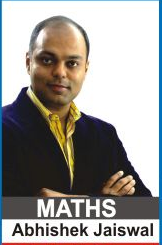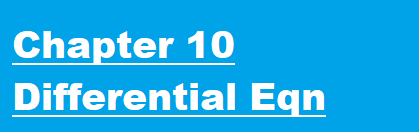Maths Lectures by Abhishek Jaiswal (B.Tech IIT Roorkee) for class 12th Students. The lectures cover theory both for CBSE and Competetive Examinations.

For Queries and Live online batches contact 9312922770.Lecture 1 | Differential Equations | Introduction

Lecture 2 | Differential Equations | Formation of Diffrential Equation

Lecture 3 | Differential Equations | Solution of Diffrential Equations

Lecture 4 | Differential Equations

Lecture 5 | Differential Equations

Lecture 6 | Differential Equations

Lecture 7 | Differential Equations | Orthogonal Trajectory

Lecture 8 | Differential Equations | Exact Differential Equation

Lecture 9 | Differential Equations

Lecture 10 | Differential Equations